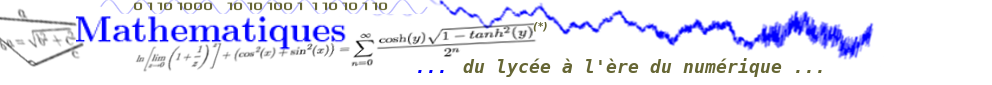🔍 Colleswhich is correct, and about which I give below the orginal text from an unknown author (as he signed himself).

# Do You Want to Become an IEEE Author?

Suppose you want to publish something that is as simple as
(E): 1 + 1 = 2
This is not very impressive. If you want your article to be accepted by IEEE reviewers, you have to be more absurd. So you could complicate the left side of the expression by using
1 = ln(e)
and
1 = cos2(x) + sin2(x)
The right hand side can be stated
2 = +∞ n=0 12n
Therefore, (E) can be expressed more "scientifically" as:
(E'): ln(e) + ( cos2(x) + sin2(x) ) = +∞ n=0 12n
which is far more impressive. However, you should not stop here. The expression can be further complicated by using
1 = cosh(y)1-tanh2(y)
and
e =  limz0 1 + 1/z n
Equation (E') may therefore be written as
ln  limz0 1 + 1/z n   + ( cos2(x) + sin2(x) ) = +∞ n=0 cosh(y)1-tanh2(y) 2n

Note: Other methods of similar nature could also be used to enhance you prestige, once you grasp the underlying principles.

The author of this "scientific" study is unknown.